TitleCollege Algebra
Tutorial 8: Simplifying Rational ExpressionsLearning Objectives

 After completing this tutorial, you should be able to: Find the domain of a rational expression. Simplify a rational expression.Introduction

 Do you ever feel like running and hiding when you see a fraction?  If so, you are not alone.  But don't fear!  Help is here!  Hey, that rhymes.  Anyway, over the next several tutorials we will be showing you several aspects of rational expressions (fractions).   In this section we will be simplifying them.  Again, we will be putting your knowledge of factoring to the test.  Factoring plays a big part of simplifying these rational expressions.  So if you feel the need to review factoring go back to Tutorial 7: Factoring Polynomials.  I think you are ready to tackle these rational expressions.Tutorial

 Rational Expression A rational expression is one that  can be written in the form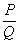where P and Q are polynomials and Q does not equal 0.

 An example of a rational expression is: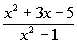Domain of a Rational Expression

 With rational functions, we need to watch out for values that cause our denominator to be 0.  If our denominator is 0, then we have an undefined value.  So, when looking for the domain of a given rational function, we use a back door approach.  We find the values that we cannot use, which would be values that make the denominator 0.Example 1:   Find all numbers that must be excluded from the domain of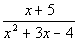.

 Our restriction is that the denominator of a fraction can never be equal to 0.  So to find what values we need to exclude, think of what value(s) of x, if any, would cause the denominator to be 0.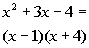*Factor the den.

 This give us a better look at it.  Since 1 would make the first factor in the denominator 0, then 1 would have to be excluded. Since - 4 would make the second factor in the denominator 0, then - 4 would also have to be excluded.

 Fundamental Principle of  Rational Expressions For any rational expression, and any polynomial R, where ,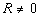, then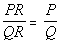In other words, if you multiply the EXACT SAME thing to the numerator and denominator, then you have an equivalent rational expression. This will come in handy when we simplify rational expressions, which is coming up next.

 Simplifying (or reducing) a  Rational Expression

 Step 1: Factor the numerator and the denominator.

 If you need a review on factoring, by all means go to Tutorial 7: Factoring Polynomials.

 Step 2: Divide out all common factors that the numerator and the denominator have.Example 2:  Simplify and find all numbers that must be excluded from the domain of the simplified rational expression: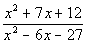.

 Step 1: Factor the numerator and the denominator AND

 Step 2: Divide out all common factors that the numerator and the denominator have.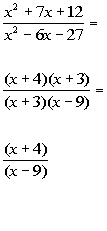*Factor the trinomials in the num. and den. *Divide out the common factor of (x + 3)   *Rational expression simplified

 To find the value(s) needed to be excluded from the domain, we need to ask ourselves, what value(s) of x would cause our denominator to be 0? Looking at the denominator x - 9, I would say it would have to be x = 9.  Don’t you agree? 9 would be our excluded value.Example 3:  Simplify and find all numbers that must be excluded from the domain of the simplified rational expression: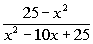.

 Step 1: Factor the numerator and the denominator AND

 Step 2: Divide out all common factors that the numerator and the denominator have.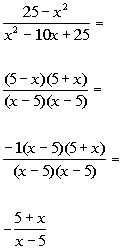*Factor the diff. of squares in the num. and  *Factor the trinomial in the den.   *Factor out a -1 from (5 - x)   *Divide out the common factor of (x - 5) *Rational expression simplified

 Note that 5 - x and x - 5 only differ by signs, in other words they are opposites of each other.  In that case, you can factor a -1 out of one of those factors and rewrite it with opposite signs, as shown in line 3 above.   To find the value(s) needed to be excluded from the domain, we need to ask ourselves, what value(s) of x would cause our denominator to be 0? Looking at the denominator x - 5, I would say it would have to be x = 5.  Don’t you agree? 5 would be our excluded value.Practice Problems

 These are practice problems to help bring you to the next level.  It will allow you to check and see if you have an understanding of these types of problems. Math works just like anything else, if you want to get good at it, then you need to practice it.  Even the best athletes and musicians had help along the way and lots of practice, practice, practice, to get good at their sport or instrument.  In fact there is no such thing as too much practice. To get the most out of these, you should work the problem out on your own and then check your answer by clicking on the link for the answer/discussion for that  problem.  At the link you will find the answer as well as any steps that went into finding that answer.Practice Problem 1a: Find all numbers that must be excluded from the domain of the given rational expression.

 1a.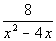(answer/discussion to 1a)Practice Problems 2a - 2b: Simplify and find all numbers that must be excluded from the domain of the simplified rational expression.

 2a.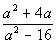(answer/discussion to 2a) 2b.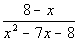(answer/discussion to 2b)Need Extra Help on these Topics?

The following are webpages that can assist you in the topics that were covered on this page:

 http://www.wtamu.edu/academic/anns/mps/math/mathlab/int_algebra/int_alg_tut32_multrat.htm The beginning of this webpage goes through how to simplify a rational expression. http://www.purplemath.com/modules/rtnldefs.htm This website helps with simplifying rational expressions.

Go to Get Help Outside the Classroom found in Tutorial 1: How to Succeed in a Math Class for some more suggestions.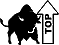Videos at this site were created and produced by Kim Seward and Virginia Williams Trice.
Last revised on Dec. 14, 2009 by Kim Seward.
All contents copyright (C) 2002 - 2010, WTAMU and Kim Seward. All rights reserved.